cancel
Showing results for
Did you mean:Helper III

## Subtotal of Amount by Person

I've been researching this all day and have had no luck.

I am dealing with employee expenses and I am connected via Direct Query.  I need to identify every employee with total expenses over \$800 which means my measure needs to be able to filtered to > \$800.  Everything I have tried only gives me individual transactions over \$800, but I need the total by person that is over \$800.

Thoughts?

Data Table:

 Emp ID Date Amount 1 1/2/2023 \$  500.00 2 2/4/2023 \$  875.00 3 2/5/2023 \$  810.00 3 3/1/2023 \$  100.00 4 1/17/2023 \$  715.00 5 1/20/2023 \$  972.00 6 2/7/2023 \$  300.00 6 2/8/2023 \$  550.00

Desired Result:

Apply filter (to visual or not): Amt per person > \$800.00

 Emp ID Amount 2 \$875 3 \$910 5 \$972 6 \$850

4 ACCEPTED SOLUTIONSSolution Sage

Hope this helps: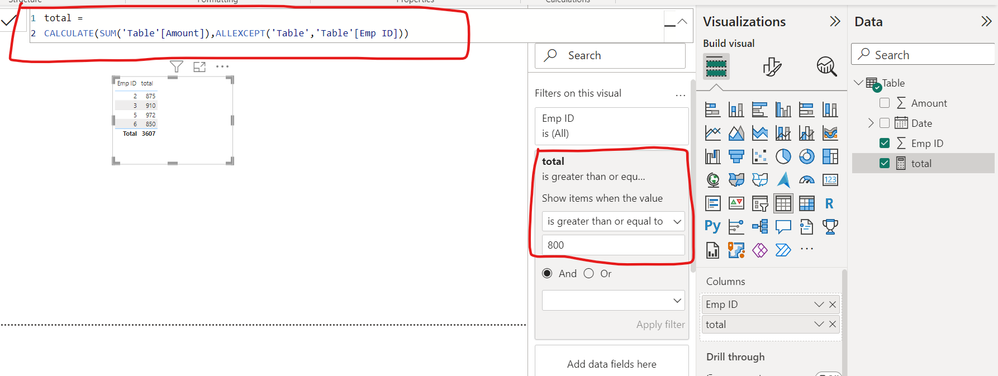Solution Sage

Thank you it makes it now more easier than 1000 words. 🙂
Please try this. The filter calculated it in the group of the Employee No.:

``
`Filter = VAR _t = CALCULATE(sum(Expenses[Amount]),ALLEXCEPT(Expenses,Expenses[Emp No]))Return _t`
You can it change it easy to a Filter for the filter pane:
`Filter = VAR _t = CALCULATE(sum(Expenses[Amount]),ALLEXCEPT(Expenses,Expenses[Emp No]))RETURN IF(_t>800,1,0)`
You can than choose "equal or greater than" 1.

Did it help?Solution Sage

My fault by copying:

`Filter = VAR _t = CALCULATE(sum(Expenses[Amount]),ALLEXCEPT(Expenses,Expenses[Emp No]))RETURN IF(_t>800,1,0)`Solution Sage

Total Exp:

``````Total Exp =
var emp = VALUES(Expenses[Emp No])
return
SUMX(
SUMMARIZE(
FILTER(ALLSELECTED(Expenses),Expenses[Emp No] in emp),
Expenses[Emp No],
"Total",SUM(Expenses[Amount])
),
[Total]
)``````

Result:
You can put it on visual level to work on aggregated items.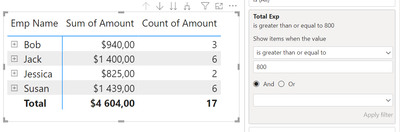Or add more columns to see details.29 REPLIES 29Solution Sage

Total Exp:

``````Total Exp =
var emp = VALUES(Expenses[Emp No])
return
SUMX(
SUMMARIZE(
FILTER(ALLSELECTED(Expenses),Expenses[Emp No] in emp),
Expenses[Emp No],
"Total",SUM(Expenses[Amount])
),
[Total]
)``````

Result:
You can put it on visual level to work on aggregated items.Or add more columns to see details.Helper III

Thank you!  This also worked for me (for all the future people searching for answers!)Solution Sage

Make a example (sample data) how this related tables are in connection with the main table (amount) and i can check it to find a general and flexible solution.Helper III

(Now I cannot get your measure to work at all - 😩 )Solution Sage

Thank you it makes it now more easier than 1000 words. 🙂
Please try this. The filter calculated it in the group of the Employee No.:

``
`Filter = VAR _t = CALCULATE(sum(Expenses[Amount]),ALLEXCEPT(Expenses,Expenses[Emp No]))Return _t`
You can it change it easy to a Filter for the filter pane:
`Filter = VAR _t = CALCULATE(sum(Expenses[Amount]),ALLEXCEPT(Expenses,Expenses[Emp No]))RETURN IF(_t>800,1,0)`
You can than choose "equal or greater than" 1.

Did it help?Helper III

Trying it all now.  The first one works beautifully (like, literally excited about it!!!).  The second, I'm getting "The syntax for 'IF' is in correct."

I cannot thank you enough!!Solution Sage

My fault by copying:

`Filter = VAR _t = CALCULATE(sum(Expenses[Amount]),ALLEXCEPT(Expenses,Expenses[Emp No]))RETURN IF(_t>800,1,0)`Helper III

Any idea why the measure above would stop working? When I try to add as a filter now, my table visual errors out.Helper III

Thank you‼️‼️‼️Solution Sage

Please set @Padycosmos post also as a solution, I see he mentioned it some days before 🙂Solution Sage

Hope this helps:Helper III

Thank you for your reply!  This gave me the same total on every line. ☹️Helper III

Using a matrix, if I only have Emp ID and Name in the Rows and (Sum of)"Amount" in the values, then the measure works like a charm.  But, if I add date or any other details, it limits the "Transaction Amount" to \$800+.  I have one person that has 28 expenses that make up a total of \$2,225.03, but if I try to get to the detail, I can't.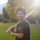Impactful Individual

Do you need a table like that or need a visual showing filtered employees?

Regardless, you can do the followings.

1. Filter in a visual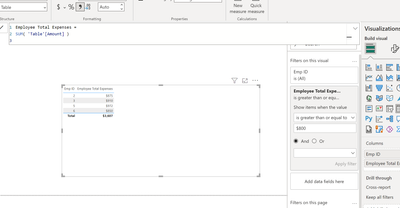2. Filtered employees table

``````Filtered Emplpoyees =
FILTER(
SUMMARIZE(
'Table',
'Table'[Emp ID]
),
[Employee Total Expenses] >= 800
)``````Helper III

Thank you for your response.  When I add the Total to a visual filter, it just gives me the individual submisions made for \$800+, not the total.

I tried the table but clearly do not have it set up correctly - would this measure be added as a filter or a column in a Matrix?  Sorry - trying to determine how to use.Impactful Individual

1. Build the measure in my screenshot I attached previously

2. Bring in EmpID into a table/matrix visual

3. Bring in the measure into the visual

4. The filter on that measure has been added to the visual

5. Filter greater than 800 in the filter

Does this help...?Helper III

I am trying to get this to work. Direct Query with a TON of data - trying to filter it down so it will refresh in a timely manner at least for testing purposes.Solution Sage
Try this: You have to change the table name.

measure =
Var _t = SUMMARIZE(ppl,ppl[Emp ID],"_sum",sum(ppl[Amount]))
RETURN sumx(FILTER(_t,[_sum]>800),[_sum])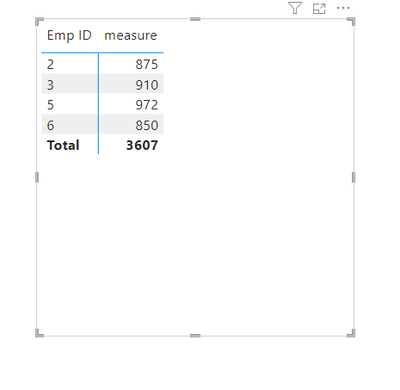Make the post as a solution if it was helpfull.Helper III

Thiis works, until I try to add a filter to remove the blanks (ie. employees whose expenses are not >\$800).  I will keep playing with it though.  Thank you for your reply!Solution Sage

let us know if you need more help. mark a or two post as solution. 🙂